# Precalculus : Find the value of the sine or cosine functions of an angle given a point on its terminal side

## Example Questions

### Example Question #1 : Find The Value Of The Sine Or Cosine Functions Of An Angle Given A Point On Its Terminal Side

What is the sine of an angle if a point on the terminal side of the angle is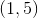?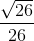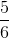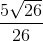Explanation:

Given the point on the coordinate plane, the origin to this point can be computed by the Pythagorean Theorem.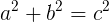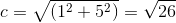The hypotenuse of the right triangle formed by the origin and the point is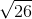.

The length of the triangle is 1 unit, and the height of the triangle is 5.

Sine of an angle is opposite side divided by the hypotenuse.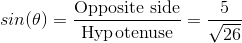Rationalize the denominator.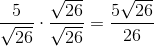### Example Question #1 : Find The Value Of The Sine Or Cosine Functions Of An Angle Given A Point On Its Terminal Side

Find the secant value of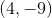if it's a point on the terminal side of an angle in standard position.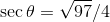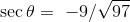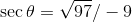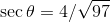Explanation:

First, use the Pythagorean Theorem to solve for all the sides of the triangle. You know that the adjacent side is 4 units long, and the opposite side is -9 units long.

Using the Pythagorean Theorem, you should get a hypotenuse of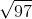.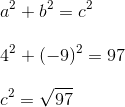Secant is defined as hypotenuse/opposite.

Thus, giving you an answer of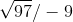.

### Example Question #1 : Find The Value Of The Sine Or Cosine Functions Of An Angle Given A Point On Its Terminal Side

Find the sine value of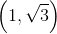if it is a point on the terminal side of an angle in standard position.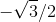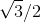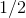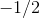Explanation:

This is a 30-60-90 triangle. A 30-60-90 triangle will have leg lengths of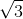and 1 and a hypotenuse of 2. To find the sin value, you need to divide the opposite leg length with the hypotenuse (opposite/hypotenuse). Thus, giving you.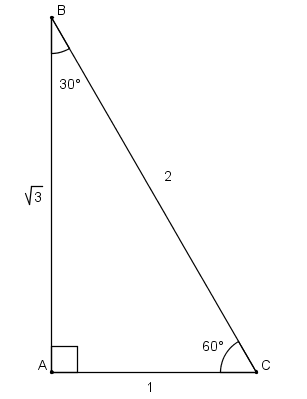### Example Question #1 : Find The Value Of The Sine Or Cosine Functions Of An Angle Given A Point On Its Terminal Side

If you are able to solve for the sine and cosine of an angle given a point on its terminal side, you have enough information to also solve for its tangent.

Which of the following best describes the validity of the above statement?

The statement is true in some cases, but not all.

The statement is true in all cases.

The statement is false in all cases.

The statement is true in all cases.

Explanation:

Recall the formula for tangent is.  If we have enough information to solve for sine and cosine, then we have the values of the opposite and adjacent of the angle.

### Example Question #1 : Find The Value Of The Sine Or Cosine Functions Of An Angle Given A Point On Its Terminal Side

The method of solving for trigonometric functions of an angle given a point on its terminal side only works for acute angles.

Which of the following statements best describes the validity of the statement above?

The statement is false

The statement is true

The statement is false

Explanation:

Consider the figure below.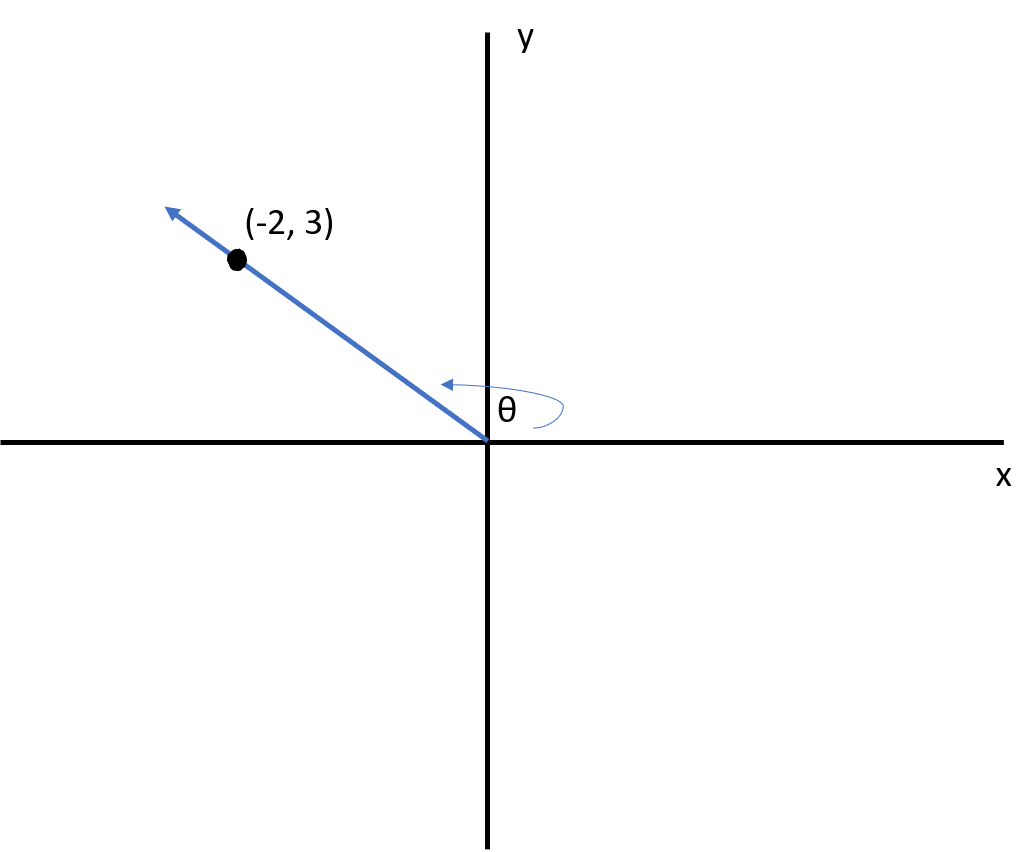We can form a triangle by dropping a line down from the point (-2,3) perpendicular to the

x axis.  Now we have right triangle that has a leg that is 3 units high and a base that is 2

units long.  Now we can use the Pythagorean Theorem to solve for the hypotenuse.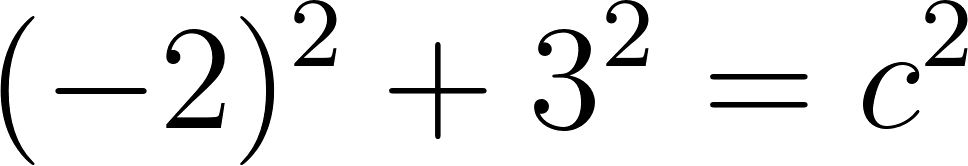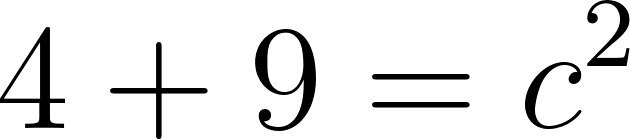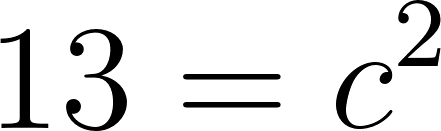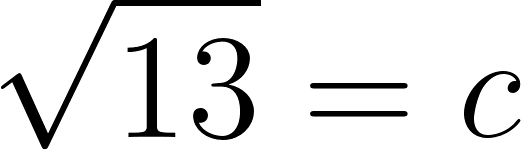We can solve for sine and cosine now.  Use the fact that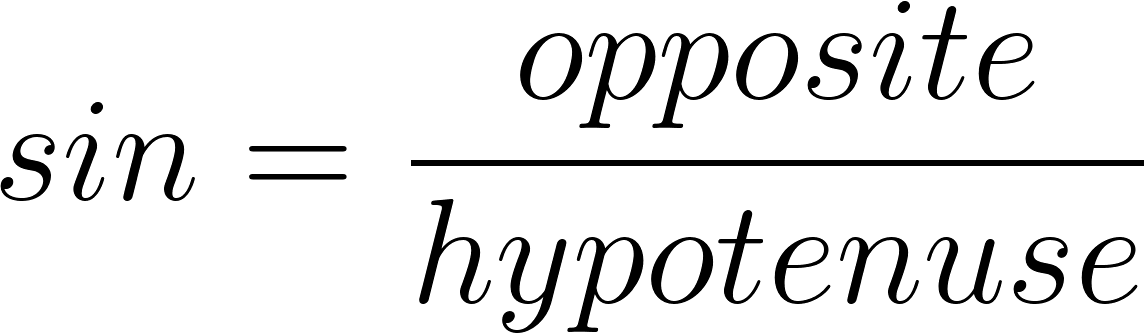.  The opposite side of the angle in this case is the leg that is 3 units high.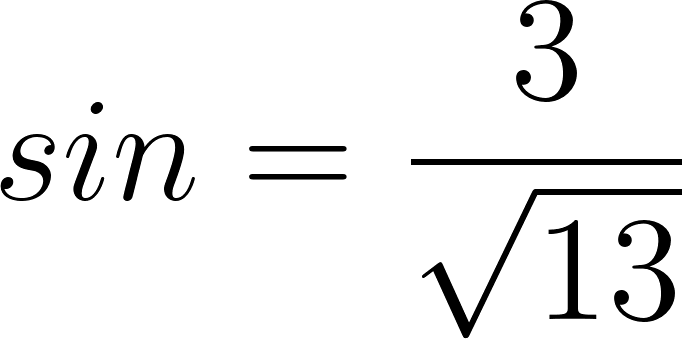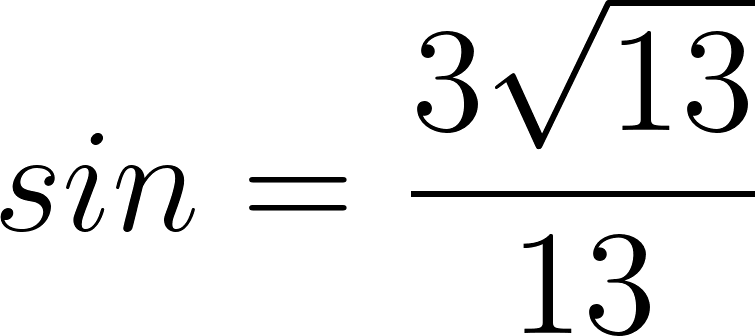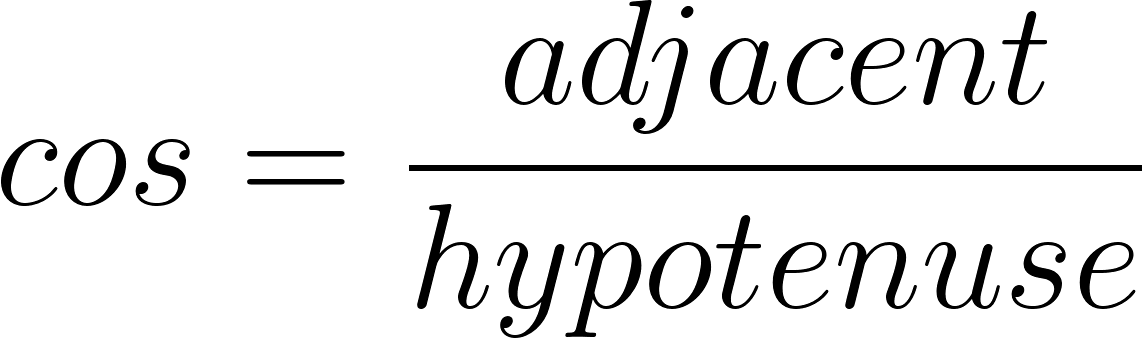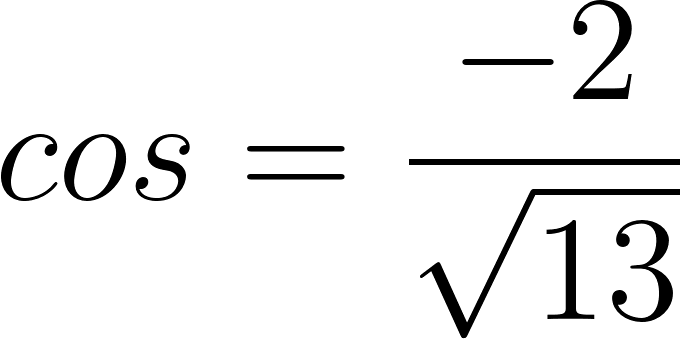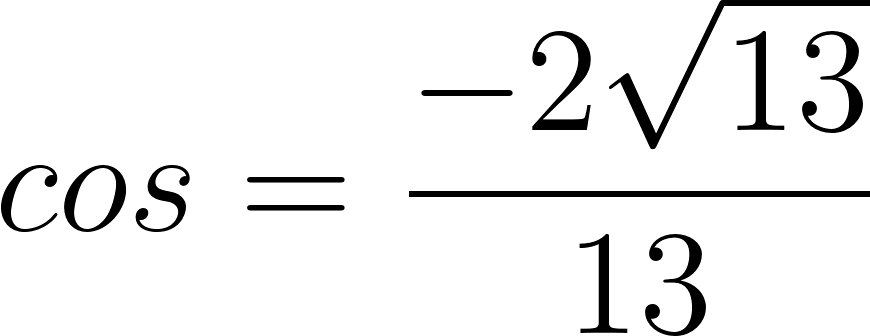So even though our angle was obtuse, we can still use the same method.

### Example Question #2 : Circular Functions

Given that the cosine of an angle is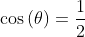, what is the height of the triangle formed by this?  (i.e. the terminal point for this angle is (1,y), solve for y).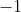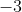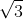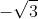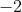### Example Question #1 : Circular Functions

Find the value of the cosine of an angle given that the point on the terminal side of the angle is (3,4).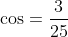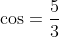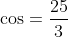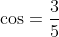Explanation:

Using the point (3,4), we can see that this forms a right triangle that has a base that is 3 units in length and an adjoining leg that is 4 units high.  We are able to find the hypotenuse of this triangle using the Pythagorean Theorem.  This will give us the distance of the point (3,4) to the origin.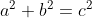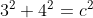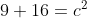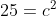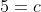And so the hypotenuse of this triangle (the distance from our point we are working with to the origin), is 5 units long.  Recall that when using cosine for right triangles, cosine represents the following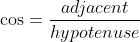So if we are considering the angle formed by the x-axis and our hypotenuse, the adjacent side would be the base of our triangle; 3 units.### Example Question #5 : Find The Value Of The Sine Or Cosine Functions Of An Angle Given A Point On Its Terminal Side

Find the sine and cosine of the following angle.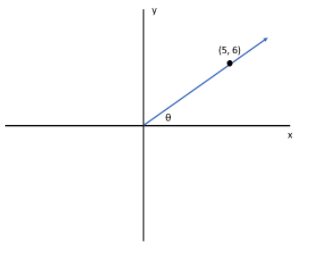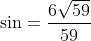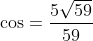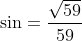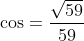Explanation:

We see that the point on the terminal side is (5,6).  So we know that with this point a right triangle is formed with a base that is 5 units long, and a leg that is 6 units high.  We can use the Pythagorean Theorem to solve for the hypotenuse that is formed by this triangle and this will tell us the distance of the point from the origin.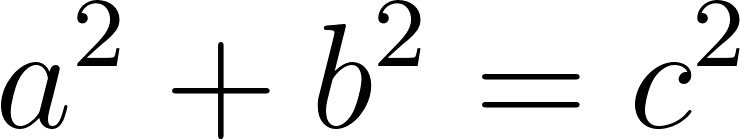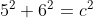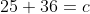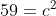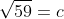Let’s solve for sine first.  When working with right triangles recall that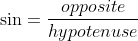and we are considering the angle formed by the x-axis and the hypotenuse.  So the opposite side is the leg that is 6 units high.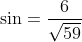We can solve for cosine if we recall that.  Our adjacent side would be the base that is 5 units long.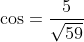### All Precalculus Resources